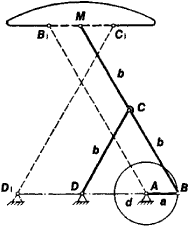# Chebyshev Parallel Motion

## Chebyshev Parallel Motion

a linkage, proposed by P. L. Chebyshev in 1868, such that some point on it describes a nearly straight line.

A straight-line mechanism, the Chebyshev parallel motion is a four-bar linkage ABCD (Figure 1) in which the lengths of the links satisfy the relation 3da = 2b. An increase in AB results in an increase in the length of the approximately rectilinear segment of the path traced by point M; at the same time, however, the deviation from a true straight line also increases.Figure 1. Chebyshev parallel motion

When in middle position, the Chebyshev parallel motion shown by solid lines in Figure 1 is reminiscent of the Greek letter lambda (λ); for this reason, it is referred to as lambda shaped. Chebyshev also proposed the variant AB1C1D1 which is shown in Figure 1 by broken lines. In this crossed-bar mechanism, the path of M coincides with the path of the same point in the lambda-shaped mechanism, and the lengths of the links satisfy the following relations: AB1 = ClD1 = 2b, B1C12a, BlMa. and AD1 = 2d. In another variant of the Chebyshev parallel motion, the angle between the lines CB and CM is not 180°.

The Chebyshev parallel motion is employed in devices for obtaining rectilinear motion of a point without the use of guides.

### REFERENCE

Chebyshev, P. L. “Ob odnom mekhanizme.” Poln. sobr. soch., vol. 4. Moscow-Leningrad, 1948.

N. I. LEVITSKII

Site: Follow: Share:
Open / Close